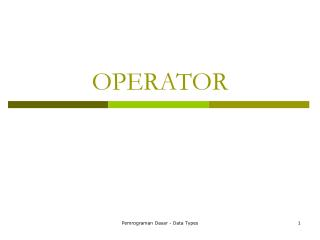DownloadDownload PresentationOPERATOR

# OPERATOR

Télécharger la présentation## OPERATOR

- - - - - - - - - - - - - - - - - - - - - - - - - - - E N D - - - - - - - - - - - - - - - - - - - - - - - - - - -
##### Presentation Transcript

1. OPERATOR Pemrograman Dasar - Data Types

2. Arithmetic operator • + - * / • / operator denotes integer division if both arguments are integer and floating-point otherwise. • Modulo operator is denoted by % (mod) • Example • 15 / 2 = 7 • 15 % 2 = 1 • 15,0 / 2 = 7,5 • Integer division by 0 raises exception • Floating-point division by 0 yields infinite or NaN (Not a number) • X += 4; is equivalent to x = x+4; Pemrograman Dasar - Data Types

3. Increment and Decrement Operator • x++, x--, ++x, --x • Be careful with the prefix and postfix form. int m = 7; Int n = 7; Int a = 2 * ++m; // now a is 16, m is 8 int b = 2 * n++; // now b is 14, n is 8 • x++ is called increment operator (x=x+1) • x-- is called decrement operator (x=x-1) Pemrograman Dasar - Data Types

4. Relational and boolean operator • To test equality, Java uses double equal signs, ==, for example 3==7 is false • Inequality is denoted by != sign, for example 3 !=7 is true • < is for less than • > is for greater than • <= is less than or equal • >= is greater than or equal • && is for ‘and’ • || is for ’or’ • ! is negation operator • Java supports ternary operator ‘?’ . The expression condition ? ex1 : ex2 evaluates to ex1 if the condition is true, to ex2 if the condition is false. E.g. x < y ? x : y gives the smaller number of x and y. Pemrograman Dasar - Data Types

5. Bitwise Operator • & (and), | (or), ^ (xor), ~ (not) • These operators work on bit patterns. • E.g int n = 17; Int fourthBitFromRight = (n & 8)/ 8; • gives a one if the fourth bit from right in the binary representation of n is one, and a zero if not. Pemrograman Dasar - Data Types

6. Mathematical Function and Constant • Math class provides mathematical function and constants • Math.sqrt(); Math.pow(x,a); // xa double x = 4; double y = Math.sqrt(x); System.out.println(y); • Notes • There is a difference betweem sqrt() method and println() method. The println() method operates on an object System.out and has second parameter ‘y’ to be printed. But the sqrt() method in the Math class does not operates on any object. It has single parameter x. Such method is called as static method Pemrograman Dasar - Data Types

7. Mathematical funcstion and constant (cont) • Math class suplies also trigonometric functions • Math.sin • Math.cos • Math.tan • Math.atan • Eponential and log • Math.exp • Math.log • Two constants • Math.PI • Math.E Pemrograman Dasar - Data Types

8. Cast • Convert a type o variable to another type • The syntax for casting is to give the target type in parentheses,followed by the variable name. • E.g. • double x = 9,997; • int y = (int)x; • So, the variable y hasthe value 9, as casting a floating-point value to an integer discards the fractional part. Pemrograman Dasar - Data Types

9. Pemrograman Dasar - Data Types